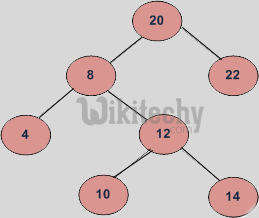# Lowest Common Ancestor in a Binary Search Tree

Python Program - Lowest Common Ancestor in a Binary Search Tree - Data Structure - write a c program to find the Lowest Common Ancestor (LCA).

Python Program Lowest Common Ancestor in Binary Search Tree:

Given values of two nodes in a Binary Search Tree, write a Python program to find the Lowest Common Ancestor (LCA). You may assume that both the values exist in the tree.

The function prototype should be as follows:

``` struct node *lca(node* root, int n1, int n2)
n1 and n2 are two given values in the tree with given root.```

For example, consider the BST in diagram, LCA of 10 and 14 is 12 and LCA of 8 and 14 is 8.

### Following is definition of LCA from Wikipedia:

Let T be a rooted tree. The lowest common ancestor between two nodes n1 and n2 is defined as the lowest node in T that has both n1 and n2 as descendants (where we allow a node to be a descendant of itself).

The LCA of n1 and n2 in T is the shared ancestor of n1 and n2 that is located farthest from the root. Computation of lowest common ancestors may be useful, for instance, as part of a procedure for determining the distance between pairs of nodes in a tree: the distance from n1 to n2 can be computed as the distance from the root to n1, plus the distance from the root to n2, minus twice the distance from the root to their lowest common ancestor. (Source Wiki)

If we are given a BST where every node has parent pointer, then LCA can be easily determined by traversing up using parent pointer and printing the first intersecting node.

We can solve this problem using BST properties. We can recursively traverse the BST from root. The main idea of the solution is, while traversing from top to bottom, the first node n we encounter with value between n1 and n2, i.e., n1 < n < n2 or same as one of the n1 or n2, is LCA of n1 and n2 (assuming that n1 < n2). So just recursively traverse the BST in, if node’s value is greater than both n1 and n2 then our LCA lies in left side of the node, if it’s is smaller than both n1 and n2, then LCA lies on right side. Otherwise root is LCA (assuming that both n1 and n2 are present in BST)

READ  C program to check whether input alphabet is a vowel or not

### Implementation of Python Program:

Python Programming
``````# A recursive python program to find LCA of two nodes
# n1 and n2

# A Binary tree node
class Node:

# Constructor to create a new node
def __init__(self, data):
self.data = data
self.left = None
self.right = None

# Function to find LCA of n1 and n2. The function assumes
# that both n1 and n2 are present in BST
def lca(root, n1, n2):

# Base Case
if root is None:
return None

# If both n1 and n2 are smaller than root, then LCA
# lies in left
if(root.data > n1 and root.data > n2):
return lca(root.left, n1, n2)

# If both n1 and n2 are greater than root, then LCA
# lies in right
if(root.data < n1 and root.data < n2):
return lca(root.right, n1, n2)

return root

# Driver program to test above function

# Let us construct the BST shown in the figure
root = Node(20)
root.left = Node(8)
root.right = Node(22)
root.left.left = Node(4)
root.left.right = Node(12)
root.left.right.left = Node(10)
root.left.right.right = Node(14)

n1 = 10 ; n2 = 14
t = lca(root, n1, n2)
print "LCA of %d and %d is %d" %(n1, n2, t.data)

n1 = 14 ; n2 = 8
t = lca(root, n1, n2)
print "LCA of %d and %d is %d" %(n1, n2 , t.data)

n1 = 10 ; n2 = 22
t = lca(root, n1, n2)
print "LCA of %d and %d is %d" %(n1, n2, t.data)

# This code is contributed by Nikhil Kumar Singh(nickzuck_007)``````

### Output:

```LCA of 10 and 14 is 12
LCA of 14 and 8 is 8
LCA of 10 and 22 is 20```

Time complexity of above solution is O(h) where h is height of tree.

Also, the above solution requires O(h) extra space in function call stack for recursive function calls. We can avoid extra space using iterative solution.#### Venkatesan Prabu

Wikitechy Founder, Author, International Speaker, and Job Consultant. My role as the CEO of Wikitechy, I help businesses build their next generation digital platforms and help with their product innovation and growth strategy. I'm a frequent speaker at tech conferences and events.

X